# QRectF¶

The `QRectF` class defines a rectangle in the plane using floating point precision. More## Detailed Description¶

A rectangle is normally expressed as a top-left corner and a size. The size (width and height) of a `QRectF` is always equivalent to the mathematical rectangle that forms the basis for its rendering.

A `QRectF` can be constructed with a set of left, top, width and height coordinates, or from a `QPointF` and a `QSizeF` . The following code creates two identical rectangles.

```r1 = QRectF(100, 200, 11, 16)
r2 = QRectF(QPoint(100, 200), QSize(11, 16))
```

There is also a third constructor creating a `QRectF` from a `QRect` , and a corresponding `toRect()` function that returns a `QRect` object based on the values of this rectangle (note that the coordinates in the returned rectangle are rounded to the nearest integer).

The `QRectF` class provides a collection of functions that return the various rectangle coordinates, and enable manipulation of these. `QRectF` also provides functions to move the rectangle relative to the various coordinates. In addition there is a `moveTo()` function that moves the rectangle, leaving its top left corner at the given coordinates. Alternatively, the `translate()` function moves the rectangle the given offset relative to the current position, and the `translated()` function returns a translated copy of this rectangle.

The `size()` function returns the rectange’s dimensions as a `QSizeF` . The dimensions can also be retrieved separately using the `width()` and `height()` functions. To manipulate the dimensions use the `setSize()` , `setWidth()` or `setHeight()` functions. Alternatively, the size can be changed by applying either of the functions setting the rectangle coordinates, for example, `setBottom()` or `setRight()` .

The `contains()` function tells whether a given point is inside the rectangle or not, and the `intersects()` function returns `true` if this rectangle intersects with a given rectangle (otherwise false). The `QRectF` class also provides the `intersected()` function which returns the intersection rectangle, and the `united()` function which returns the rectangle that encloses the given rectangle and this: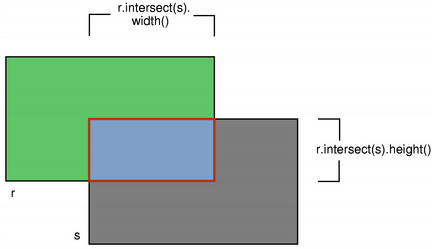`intersected()` `united()`

The `isEmpty()` function returns `true` if the rectangle’s width or height is less than, or equal to, 0. Note that an empty rectangle is not valid: The `isValid()` function returns `true` if both width and height is larger than 0. A null rectangle ( `isNull()` == true) on the other hand, has both width and height set to 0.

Note that due to the way `QRect` and `QRectF` are defined, an empty `QRectF` is defined in essentially the same way as `QRect` .

Finally, `QRectF` objects can be streamed as well as compared.

### Rendering¶

When using an `anti-aliased` painter, the boundary line of a `QRectF` will be rendered symmetrically on both sides of the mathematical rectangle’s boundary line. But when using an aliased painter (the default) other rules apply.

Then, when rendering with a one pixel wide pen the `QRectF` ‘s boundary line will be rendered to the right and below the mathematical rectangle’s boundary line.

When rendering with a two pixels wide pen the boundary line will be split in the middle by the mathematical rectangle. This will be the case whenever the pen is set to an even number of pixels, while rendering with a pen with an odd number of pixels, the spare pixel will be rendered to the right and below the mathematical rectangle as in the one pixel case.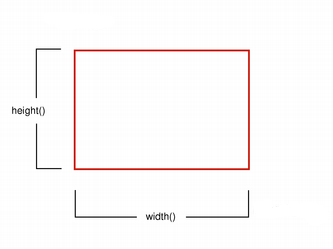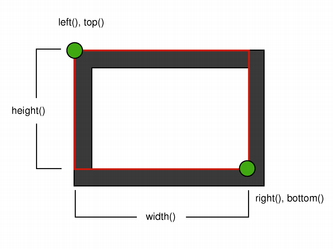Logical representation One pixel wide pen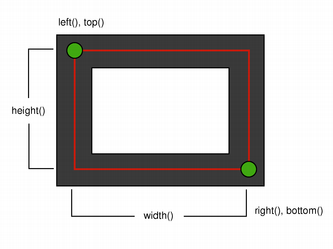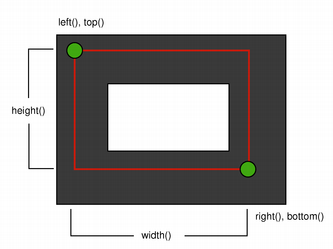Two pixel wide pen Three pixel wide pen

### Coordinates¶

The `QRectF` class provides a collection of functions that return the various rectangle coordinates, and enable manipulation of these. `QRectF` also provides functions to move the rectangle relative to the various coordinates.

For example: the `bottom()` , `setBottom()` and `moveBottom()` functions: `bottom()` returns the y-coordinate of the rectangle’s bottom edge, `setBottom()` sets the bottom edge of the rectangle to the given y coordinate (it may change the height, but will never change the rectangle’s top edge) and `moveBottom()` moves the entire rectangle vertically, leaving the rectangle’s bottom edge at the given y coordinate and its size unchanged.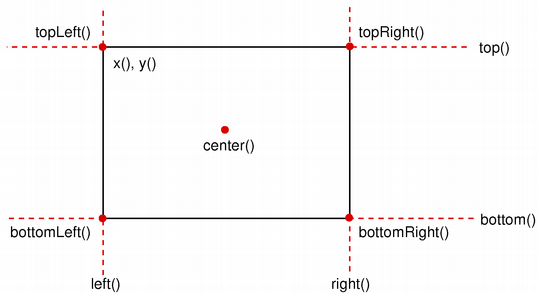It is also possible to add offsets to this rectangle’s coordinates using the `adjust()` function, as well as retrieve a new rectangle based on adjustments of the original one using the `adjusted()` function. If either of the width and height is negative, use the `normalized()` function to retrieve a rectangle where the corners are swapped.

In addition, `QRectF` provides the `getCoords()` function which extracts the position of the rectangle’s top-left and bottom-right corner, and the `getRect()` function which extracts the rectangle’s top-left corner, width and height. Use the `setCoords()` and `setRect()` function to manipulate the rectangle’s coordinates and dimensions in one go.

class `QRectF`

QRectF(topleft, bottomRight)

QRectF(topleft, size)

QRectF(rect)

QRectF(QRectF)

QRectF(left, top, width, height)

param size

`QSizeF`

param top

`qreal`

param QRectF

`QRectF`

param bottomRight

`QPointF`

param topleft

`QPointF`

param rect

`QRect`

param left

`qreal`

param width

`qreal`

param height

`qreal`

Constructs a null rectangle.

Constructs a `QRectF` rectangle from the given `QRect` `rectangle` .

Constructs a rectangle with (`x` , `y` ) as its top-left corner and the given `width` and `height` .

`PySide2.QtCore.QRectF.``__reduce__`()
Return type

`PyObject`

`PySide2.QtCore.QRectF.``__repr__`()
Return type

`PyObject`

`PySide2.QtCore.QRectF.``adjust`(x1, y1, x2, y2)
Parameters
• x1`qreal`

• y1`qreal`

• x2`qreal`

• y2`qreal`

Adds `dx1` , `dy1` , `dx2` and `dy2` respectively to the existing coordinates of the rectangle.

`PySide2.QtCore.QRectF.``adjusted`(x1, y1, x2, y2)
Parameters
• x1`qreal`

• y1`qreal`

• x2`qreal`

• y2`qreal`

Return type

`QRectF`

Returns a new rectangle with `dx1` , `dy1` , `dx2` and `dy2` added respectively to the existing coordinates of this rectangle.

`PySide2.QtCore.QRectF.``bottom`()
Return type

`qreal`

Returns the y-coordinate of the rectangle’s bottom edge.

`PySide2.QtCore.QRectF.``bottomLeft`()
Return type

`QPointF`

Returns the position of the rectangle’s bottom-left corner.

`PySide2.QtCore.QRectF.``bottomRight`()
Return type

`QPointF`

Returns the position of the rectangle’s bottom-right corner.

`PySide2.QtCore.QRectF.``center`()
Return type

`QPointF`

Returns the center point of the rectangle.

`PySide2.QtCore.QRectF.``contains`(r)
Parameters
Return type

`bool`

`PySide2.QtCore.QRectF.``contains`(x, y)
Parameters
• x`qreal`

• y`qreal`

Return type

`bool`

Returns `true` if the point (`x` , `y` ) is inside or on the edge of the rectangle; otherwise returns `false` .

`PySide2.QtCore.QRectF.``contains`(p)
Parameters
Return type

`bool`

`PySide2.QtCore.QRectF.``getCoords`()

Extracts the position of the rectangle’s top-left corner to *``x1`` and *``y1`` , and the position of the bottom-right corner to *``x2`` and *``y2`` .

`PySide2.QtCore.QRectF.``getRect`()

Extracts the position of the rectangle’s top-left corner to *``x`` and *``y`` , and its dimensions to *``width`` and *``height`` .

`PySide2.QtCore.QRectF.``height`()
Return type

`qreal`

Returns the height of the rectangle.

`PySide2.QtCore.QRectF.``intersected`(other)
Parameters

other`QRectF`

Return type

`QRectF`

Returns the intersection of this rectangle and the given `rectangle` . Note that `r.intersected(s)` is equivalent to `r & s` .`intersects()` `united()` `operator&=()`

`PySide2.QtCore.QRectF.``intersects`(r)
Parameters
Return type

`bool`

Returns `true` if this rectangle intersects with the given `rectangle` (i.e. there is a non-empty area of overlap between them), otherwise returns `false` .

The intersection rectangle can be retrieved using the `intersected()` function.

`PySide2.QtCore.QRectF.``isEmpty`()
Return type

`bool`

Returns `true` if the rectangle is empty, otherwise returns `false` .

An empty rectangle has `width()` <= 0 or `height()` <= 0. An empty rectangle is not valid (i.e., == ! `isValid()` ).

Use the `normalized()` function to retrieve a rectangle where the corners are swapped.

`PySide2.QtCore.QRectF.``isNull`()
Return type

`bool`

Returns `true` if the rectangle is a null rectangle, otherwise returns `false` .

A null rectangle has both the width and the height set to 0. A null rectangle is also empty, and hence not valid.

`PySide2.QtCore.QRectF.``isValid`()
Return type

`bool`

Returns `true` if the rectangle is valid, otherwise returns `false` .

A valid rectangle has a `width()` > 0 and `height()` > 0. Note that non-trivial operations like intersections are not defined for invalid rectangles. A valid rectangle is not empty (i.e., == ! `isEmpty()` ).

`PySide2.QtCore.QRectF.``left`()
Return type

`qreal`

Returns the x-coordinate of the rectangle’s left edge. Equivalent to `x()` .

`PySide2.QtCore.QRectF.``marginsAdded`(margins)
Parameters

margins`QMarginsF`

Return type

`QRectF`

Returns a rectangle grown by the `margins` .

`operator+=()` `marginsRemoved()` `operator-=()`

`PySide2.QtCore.QRectF.``marginsRemoved`(margins)
Parameters

margins`QMarginsF`

Return type

`QRectF`

Removes the `margins` from the rectangle, shrinking it.

`marginsAdded()` `operator+=()` `operator-=()`

`PySide2.QtCore.QRectF.``moveBottom`(pos)
Parameters

pos`qreal`

Moves the rectangle vertically, leaving the rectangle’s bottom edge at the given `y` coordinate. The rectangle’s size is unchanged.

`PySide2.QtCore.QRectF.``moveBottomLeft`(p)
Parameters

Moves the rectangle, leaving the bottom-left corner at the given `position` . The rectangle’s size is unchanged.

`PySide2.QtCore.QRectF.``moveBottomRight`(p)
Parameters

Moves the rectangle, leaving the bottom-right corner at the given `position` . The rectangle’s size is unchanged.

`PySide2.QtCore.QRectF.``moveCenter`(p)
Parameters

Moves the rectangle, leaving the center point at the given `position` . The rectangle’s size is unchanged.

`PySide2.QtCore.QRectF.``moveLeft`(pos)
Parameters

pos`qreal`

Moves the rectangle horizontally, leaving the rectangle’s left edge at the given `x` coordinate. The rectangle’s size is unchanged.

`PySide2.QtCore.QRectF.``moveRight`(pos)
Parameters

pos`qreal`

Moves the rectangle horizontally, leaving the rectangle’s right edge at the given `x` coordinate. The rectangle’s size is unchanged.

`PySide2.QtCore.QRectF.``moveTo`(p)
Parameters

Moves the rectangle, leaving the top-left corner at the given `position` .

`PySide2.QtCore.QRectF.``moveTo`(x, y)
Parameters
• x`qreal`

• y`qreal`

Moves the rectangle, leaving the top-left corner at the given position (`x` , `y` ). The rectangle’s size is unchanged.

`PySide2.QtCore.QRectF.``moveTop`(pos)
Parameters

pos`qreal`

Moves the rectangle vertically, leaving the rectangle’s top line at the given `y` coordinate. The rectangle’s size is unchanged.

`PySide2.QtCore.QRectF.``moveTopLeft`(p)
Parameters

Moves the rectangle, leaving the top-left corner at the given `position` . The rectangle’s size is unchanged.

`PySide2.QtCore.QRectF.``moveTopRight`(p)
Parameters

Moves the rectangle, leaving the top-right corner at the given `position` . The rectangle’s size is unchanged.

`PySide2.QtCore.QRectF.``normalized`()
Return type

`QRectF`

Returns a normalized rectangle; i.e., a rectangle that has a non-negative width and height.

If `width()` < 0 the function swaps the left and right corners, and it swaps the top and bottom corners if `height()` < 0.

`PySide2.QtCore.QRectF.``__ne__`(arg__2)
Parameters

arg__2`QRectF`

Return type

`bool`

`PySide2.QtCore.QRectF.``__ne__`(r2)
Parameters
Return type

`bool`

`PySide2.QtCore.QRectF.``__and__`(r)
Parameters
Return type

`QRectF`

`PySide2.QtCore.QRectF.``__iand__`(r)
Parameters
Return type

`QRectF`

`PySide2.QtCore.QRectF.``__add__`(lhs)
Parameters
Return type

`QRectF`

`PySide2.QtCore.QRectF.``__add__`(rhs)
Parameters
Return type

`QRectF`

`PySide2.QtCore.QRectF.``__iadd__`(margins)
Parameters

margins`QMarginsF`

Return type

`QRectF`

Adds the `margins` to the rectangle, growing it.

`marginsAdded()` `marginsRemoved()` `operator-=()`

`PySide2.QtCore.QRectF.``__sub__`(rhs)
Parameters
Return type

`QRectF`

`PySide2.QtCore.QRectF.``__isub__`(margins)
Parameters

margins`QMarginsF`

Return type

`QRectF`

Returns a rectangle shrunk by the `margins` .

`marginsRemoved()` `operator+=()` `marginsAdded()`

`PySide2.QtCore.QRectF.``__eq__`(arg__2)
Parameters

arg__2`QRectF`

Return type

`bool`

`PySide2.QtCore.QRectF.``__eq__`(r2)
Parameters
Return type

`bool`

`PySide2.QtCore.QRectF.``__or__`(r)
Parameters
Return type

`QRectF`

Returns the bounding rectangle of this rectangle and the given `rectangle` .

`united()` `operator|=()`

`PySide2.QtCore.QRectF.``__ior__`(r)
Parameters
Return type

`QRectF`

Unites this rectangle with the given `rectangle` .

`united()` `operator|()`

`PySide2.QtCore.QRectF.``right`()
Return type

`qreal`

Returns the x-coordinate of the rectangle’s right edge.

`PySide2.QtCore.QRectF.``setBottom`(pos)
Parameters

pos`qreal`

Sets the bottom edge of the rectangle to the given `y` coordinate. May change the height, but will never change the top edge of the rectangle.

`PySide2.QtCore.QRectF.``setBottomLeft`(p)
Parameters

Set the bottom-left corner of the rectangle to the given `position` . May change the size, but will never change the top-right corner of the rectangle.

`PySide2.QtCore.QRectF.``setBottomRight`(p)
Parameters

Set the bottom-right corner of the rectangle to the given `position` . May change the size, but will never change the top-left corner of the rectangle.

`PySide2.QtCore.QRectF.``setCoords`(x1, y1, x2, y2)
Parameters
• x1`qreal`

• y1`qreal`

• x2`qreal`

• y2`qreal`

Sets the coordinates of the rectangle’s top-left corner to (`x1` , `y1` ), and the coordinates of its bottom-right corner to (`x2` , `y2` ).

`PySide2.QtCore.QRectF.``setHeight`(h)
Parameters

h`qreal`

Sets the height of the rectangle to the given `height` . The bottom edge is changed, but not the top one.

`PySide2.QtCore.QRectF.``setLeft`(pos)
Parameters

pos`qreal`

Sets the left edge of the rectangle to the given `x` coordinate. May change the width, but will never change the right edge of the rectangle.

Equivalent to `setX()` .

`PySide2.QtCore.QRectF.``setRect`(x, y, w, h)
Parameters
• x`qreal`

• y`qreal`

• w`qreal`

• h`qreal`

Sets the coordinates of the rectangle’s top-left corner to (`x` , `y` ), and its size to the given `width` and `height` .

`PySide2.QtCore.QRectF.``setRight`(pos)
Parameters

pos`qreal`

Sets the right edge of the rectangle to the given `x` coordinate. May change the width, but will never change the left edge of the rectangle.

`PySide2.QtCore.QRectF.``setSize`(s)
Parameters

Sets the size of the rectangle to the given `size` . The top-left corner is not moved.

`PySide2.QtCore.QRectF.``setTop`(pos)
Parameters

pos`qreal`

Sets the top edge of the rectangle to the given `y` coordinate. May change the height, but will never change the bottom edge of the rectangle.

Equivalent to `setY()` .

`PySide2.QtCore.QRectF.``setTopLeft`(p)
Parameters

Set the top-left corner of the rectangle to the given `position` . May change the size, but will never change the bottom-right corner of the rectangle.

`PySide2.QtCore.QRectF.``setTopRight`(p)
Parameters

Set the top-right corner of the rectangle to the given `position` . May change the size, but will never change the bottom-left corner of the rectangle.

`PySide2.QtCore.QRectF.``setWidth`(w)
Parameters

w`qreal`

Sets the width of the rectangle to the given `width` . The right edge is changed, but not the left one.

`PySide2.QtCore.QRectF.``setX`(pos)
Parameters

pos`qreal`

Sets the left edge of the rectangle to the given `x` coordinate. May change the width, but will never change the right edge of the rectangle.

Equivalent to `setLeft()` .

`PySide2.QtCore.QRectF.``setY`(pos)
Parameters

pos`qreal`

Sets the top edge of the rectangle to the given `y` coordinate. May change the height, but will never change the bottom edge of the rectangle.

Equivalent to `setTop()` .

`PySide2.QtCore.QRectF.``size`()
Return type

`QSizeF`

Returns the size of the rectangle.

`PySide2.QtCore.QRectF.``toAlignedRect`()
Return type

`QRect`

Returns a `QRect` based on the values of this rectangle that is the smallest possible integer rectangle that completely contains this rectangle.

`PySide2.QtCore.QRectF.``toRect`()
Return type

`QRect`

Returns a `QRect` based on the values of this rectangle. Note that the coordinates in the returned rectangle are rounded to the nearest integer.

`QRectF()` `toAlignedRect()`

`PySide2.QtCore.QRectF.``top`()
Return type

`qreal`

Returns the y-coordinate of the rectangle’s top edge. Equivalent to `y()` .

`PySide2.QtCore.QRectF.``topLeft`()
Return type

`QPointF`

Returns the position of the rectangle’s top-left corner.

`PySide2.QtCore.QRectF.``topRight`()
Return type

`QPointF`

Returns the position of the rectangle’s top-right corner.

`PySide2.QtCore.QRectF.``translate`(p)
Parameters

Moves the rectangle `offset` . `x()` along the x axis and `offset` . `y()` along the y axis, relative to the current position.

`PySide2.QtCore.QRectF.``translate`(dx, dy)
Parameters
• dx`qreal`

• dy`qreal`

Moves the rectangle `dx` along the x-axis and `dy` along the y-axis, relative to the current position. Positive values move the rectangle to the right and downwards.

`PySide2.QtCore.QRectF.``translated`(p)
Parameters
Return type

`QRectF`

Returns a copy of the rectangle that is translated `offset` . `x()` along the x axis and `offset` . `y()` along the y axis, relative to the current position.

`PySide2.QtCore.QRectF.``translated`(dx, dy)
Parameters
• dx`qreal`

• dy`qreal`

Return type

`QRectF`

Returns a copy of the rectangle that is translated `dx` along the x axis and `dy` along the y axis, relative to the current position. Positive values move the rectangle to the right and down.

`PySide2.QtCore.QRectF.``transposed`()
Return type

`QRectF`

Returns a copy of the rectangle that has its width and height exchanged:

```QRectF r = {1.5, 5.1, 4.2, 2.4};
r = r.transposed(); // r == {1.5, 5.1, 2.4, 4.2}
```
`PySide2.QtCore.QRectF.``united`(other)
Parameters

other`QRectF`

Return type

`QRectF`

Returns the bounding rectangle of this rectangle and the given `rectangle` .`PySide2.QtCore.QRectF.``width`()
Return type

`qreal`

Returns the width of the rectangle.

`PySide2.QtCore.QRectF.``x`()
Return type

`qreal`

Returns the x-coordinate of the rectangle’s left edge. Equivalent to `left()` .

`PySide2.QtCore.QRectF.``y`()
`qreal`
Returns the y-coordinate of the rectangle’s top edge. Equivalent to `top()` .